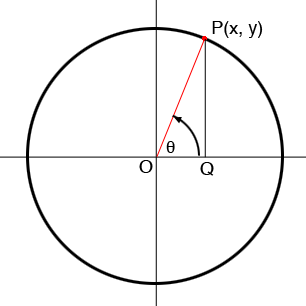SEARCH HOMEMath Central Quandaries & QueriesQuestion from Jake, a student: I was wondering what conclusions can be drawn about the trigonometric functions and how they work about the circle. Can you also please give me an explanation for it? Thank you.Hi Jake,

Draw a unit circle with centre at the origin $O$ in the Cartesian plane.Let $P$ with coordinates $(x, y)$ be a point on the circle. Measure the angle $\theta$ between the positive $X$ axis and the ray $OP$ in radians where a positive angle is measured counterclockwise. $x$ is then the cosine of the angle $\theta$ and $y$ is the sine of $\theta,$ that is

$x = \cos(\theta) \mbox{ and } y = \sin(\theta).$

This is one way to define the sine and cosine and then you can define the other trig functions in terms of sine and cosine. I drew the vertical line segment $PQ$ so that you can use the triangle $OQP$ to relate the trig functions defined using the triangle to the definition I just gave using the circle. Remember that the length of $OP$ is 1.

I hope this helps,
PennyMath Central is supported by the University of Regina and the Imperial Oil Foundation.